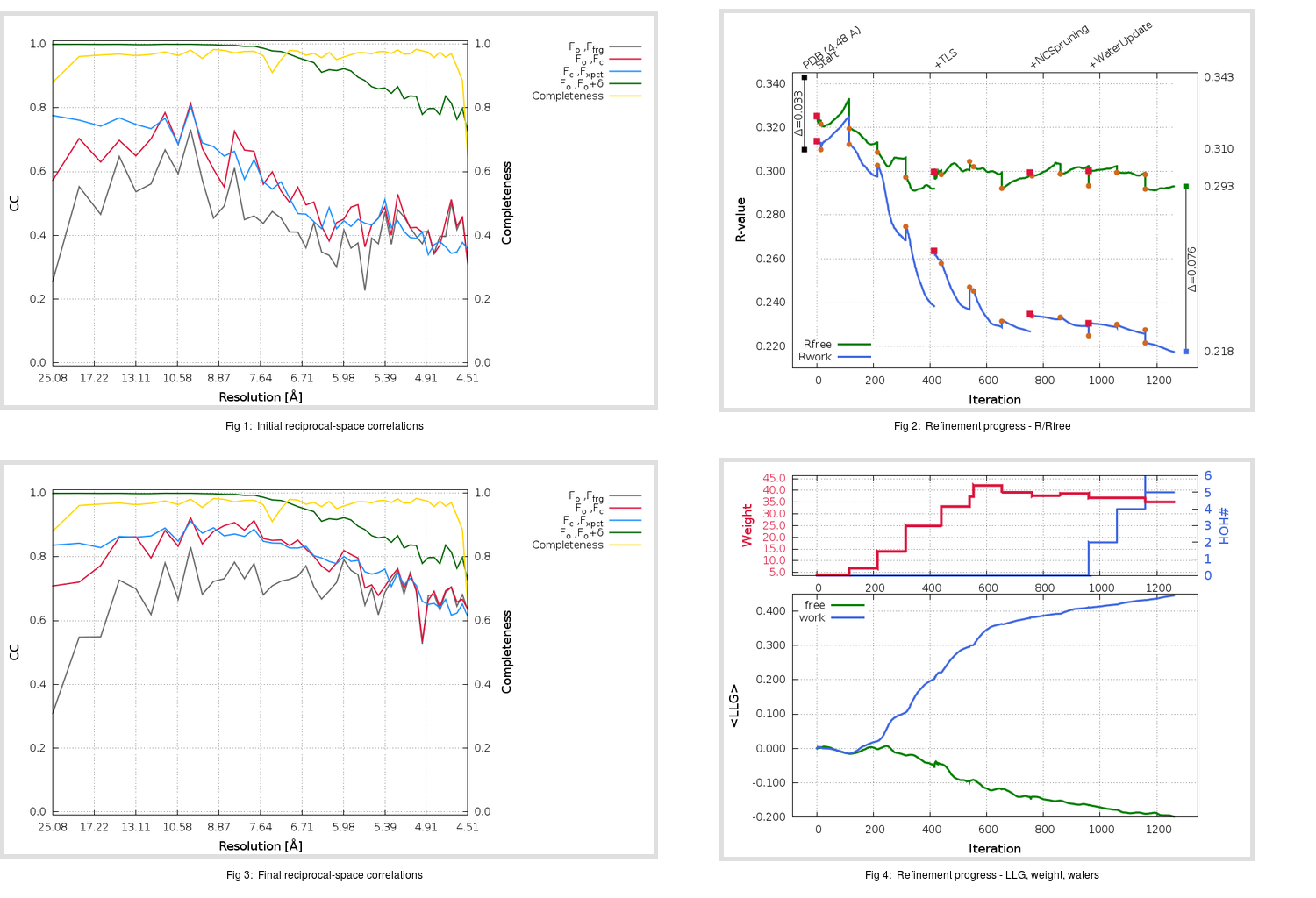Content:

## Deposited

` `
 Date deposited Date data collection Resolution R, Rfree 20180521 20160515 4.48 0.3070 0.3430

Molprobity (CCP4 7.0 version) summary:

```Ramachandran outliers =   2.02 %
favored =  92.33 %
Rotamer outliers      =   4.17 %
C-beta deviations     =     0
Clashscore            =  16.74
RMS(bonds)            =   0.0063
RMS(angles)           =   1.23
MolProbity score      =   2.67
Resolution            =   4.48
R-work                =   0.3070
R-free                =   0.3430
```

```Number of waters      =     0

<B> (all atoms) =  308.02 ( sd =   77.23 ) for       9462 non-hydrogen atoms
<B>   (protein) =  308.02 ( sd =   77.23 ) for       9462 non-hydrogen atoms
<B>     (water) =    0.00 ( sd =    0.00 ) for          0 non-hydrogen atoms
<B>    (others) =    0.00 ( sd =    0.00 ) for          0 non-hydrogen atoms

B min/max       (all non-hydrogen atoms) =  178.20 /  615.33
B min/max   (protein non-hydrogen atoms) =  178.20 /  615.33
B min/max     (water non-hydrogen atoms) =    0.00 /    0.00
B min/max     (other non-hydrogen atoms) =    0.00 /    0.00
```

## BUSTER (re-)refinement

` `

Molprobity (CCP4 7.0 version) summary:

```Ramachandran outliers =   5.17 %
favored =  77.30 %
Rotamer outliers      =  12.71 %
C-beta deviations     =     1
Clashscore            =  17.98
RMS(bonds)            =   0.0122
RMS(angles)           =   1.73
MolProbity score      =   3.36
Resolution            =   4.48
R-work                =   0.2175
R-free                =   0.2931
```

```Number of waters      =     5

<B> (all atoms) =  258.05 ( sd =   43.85 ) for       9467 non-hydrogen atoms
<B>   (protein) =  258.05 ( sd =   43.86 ) for       9462 non-hydrogen atoms
<B>     (water) =  258.76 ( sd =    0.12 ) for          5 non-hydrogen atoms
<B>    (others) =    0.00 ( sd =    0.00 ) for          0 non-hydrogen atoms

B min/max       (all non-hydrogen atoms) =  128.34 /  300.00
B min/max   (protein non-hydrogen atoms) =  128.34 /  300.00
B min/max     (water non-hydrogen atoms) =  258.58 /  258.94
B min/max     (other non-hydrogen atoms) =    0.00 /    0.00
```

Refinement progression:Results:

` `
 File Remark 5ZXV_aB_refine.01_04_refine.pdb.gz exact refinement commands are in header 5ZXV_aB_refine.01_04_refine.mtz.gz including original deposited data and several re-refinement map coefficients 5ZXV_aB_refine.01_04_BUSTER_model.cif.gz including any non-standard compound restraints 5ZXV_aB_refine.01_04_BUSTER_refln.cif.gz